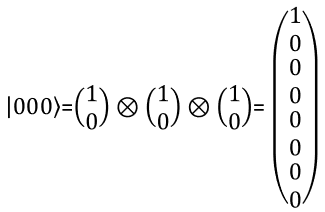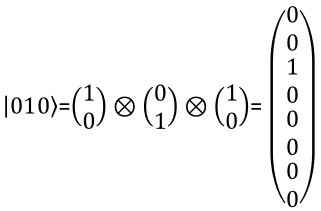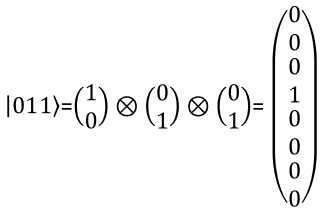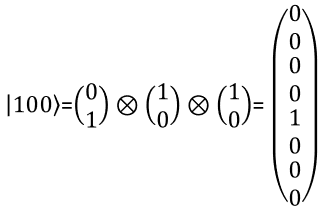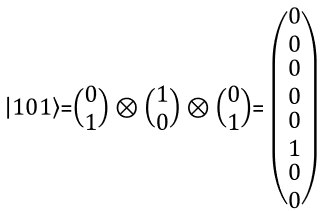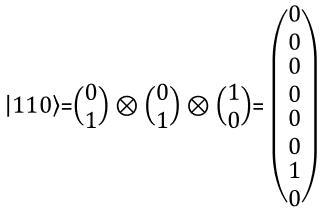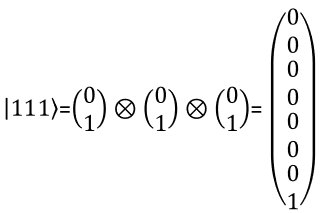Quamtum Computing                                                                        Home : www.sharetechnote.com Qbit/Qubit   What is Qbit ? It stands for Quantum bit, but I am not going to explain on what is Qbit in this page. For this kind of explanation, I think some of the video in the video link page would do better job. Do not expect to understand the concept just by watching one or two videos. Try to find as many different ones as possible and watch all of them rather than watching only one video so over and over.   For now, just take that Qbit is the basic processing unit in Quamtum computing as the basic processing unit in ordinary computing (classical computing) is called a bit.   The critical differece between Qbit and ordinary Bit is that Qbit acts according to fundamental nature of quantum mechanics called Superposition and Entanglement (don't ask me what these are -:).   Again there are a lot of videos trying to explain on these concept).       Qbit vs Classical Bit   As mentioned before, it would be very difficult to explain on the definition of Qbit(Qubit) verbally. Even if it is possible, I don't think I can capable of explaining it better than what others do in many blog and youTube. What I am trying to do here is just to give you a few keywords that you can begin with or simply using it as a keyword when you do googling. Qbit is the basic unit in Quantum Computing and it has following basic nature of quamtum mechanics and this is the main difference between Qbit and Bit (The classical bit : the unit that we use in our regular computing).   Superposition : The property of a bit being present simultaneously in multiple different states. Simply put, the bit 0 and 1 can coexists simultaneously. Entanglement : A special property of correlation in quantum partitle in which a state of a partitle automatically determines the state of another particle.          How to represent a Spin ?   Everybody would now know how a bit is represented in ordinary computing. It is represented by two numbers 0 and 1. But is this 0 and 1 a real physical entity ?  Now you also know the 0 and 1 is not the physical entity. When they are talking about physical entities for the bit 0 or 1, some people try to explain it with some physical electric switch On and Off. or if they want to go a little bit deeper into technology they may talk about some kind of transistor with the operation voltage of 5V or 3.3V or 1.8V etc. However they are trying to explain on the physical entities to the logical bits 0 and 1, it would be almost impossible to get yourself fully understood about it unless you are a physist working specifically on this area. There are still so many physists and engineers working only in the area of implemeting the physical entities for the logical bit 0 and 1. But almost everybody working with computer programming for hobby or for job can do their job just based on the concept of logical bit 0 or 1 without much or almost no knowledge on the physical entities of the logical bit. The reason why I am talking about this is for those people who claim that I cannot learn any new technologies without completely understanding the fundamental knowledge on how it works. I used to a person of this kind and still try to all my best to understand a new technology as much in fundamentals as possible. So I was reluctant to trying Quamtum computing (Quantum Programming) without mastering Quatum Theory/Quantum Mechanics. As time goes on, I started realizing that it is as if I say "I say I would not try to learn C programing or Python programming unless I master the study of solid state physics on transister". What I am trying to say is... what I am trying to explain (tyring to learn at the same time) in my note is mostly about logical entities of a Quamtum bit which can be understood without the deep knowledge of physics theory behind it. The only remaining hurdle is that you still need to get familiar with a little bit of math at this stage. I believe that the time will come in the future when even this kind of simple math would not be required in quantum computing, but for now (Dec 2019) I don't think we can avoid some simple math since quantum computing is at such an early stage like early stages of ordinary computing programming in machine code or assembly code.   Now let's get down to the job talking about Qbit. As we make an analogy between logical bits and physical entities (like transistor voltage), they have invented a logical concept in quantum mechnics which is called a spin. There are two types of spin. One is called Upspin representing spining in counter clockwise and the other one is called downspin representing spinining in clockwise. Is there any physical entities like an electron or an atom spinning around ? Physist say No. They say 'spin' is a kind of logical concept representing the nature of a quamtum particle and they can be explained only in mathematical model. It does not really mean that we can see an electron or an atom spinning in front of our eyes. So for now, let's just accept that a quamtum bit is represented by two different spin called upspin and downspin.   Just accepting all of the logical concept as given to us, let's look further into how a quamtum bit is represented. I illustrated various way of representing a quantum bit as shown below.   The left most one is how upspin is represented graphically. The next strange symbol | >. This symbol is called Bra-ket. |0> represents the quatom bit 0. And then you see a vector. This vector also represent the quatum bit 0. The last one also reprents the quatum bit 0 in a probability function and in a coordinate system. I will talk a little bit detail about this later.The left most one is how downspin is represented graphically. |1> represents the quatom bit 1. And then you see a vector. This vector also represent the quatum bit 1. The last one also reprents the quatum bit 1 in a probability function and in a coordinate system. I will talk a little bit detail about this later.The horizontal axis of the coordinate system indicates the probability of the bit existing as a quatum bit 0 and the vertical axis indicate the probability of the bit existing as a quamtum bit 1 as indicated below.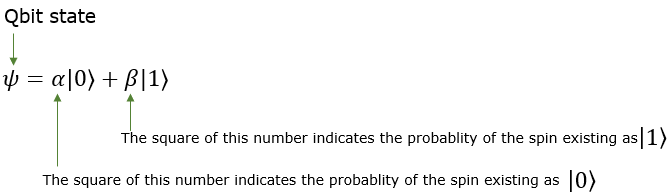This probability function (Quamtum State function) is represented in a vector notation as follows. You should be very familiar with this notation because this will be the basis for all the operators in quantum programming. Make practice as much as possible on translating a state vector in plain language.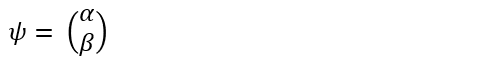The number of this vector (defined as below) should be 1 as below.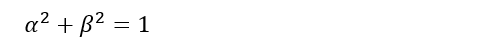If alpha and beta is complex numbers, the total probability is calculated as follows (i.e, multiplying each value with its complex conjugate).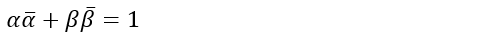Since the norm of the state vector is 1, all the possible states represted by the state vector should all be on the peripheral of a unit circle. Some of the most commonly used state vector is mapped onto a unit circle as below. Try to translate each location marked here in plain language. For example, [1/Sqr(2) 1/Sqr(2)] indicates that the bit would be existing as the quantum bit 0 with the probability of 50% and be existing as the quantum bit 1 with the probability of 50%.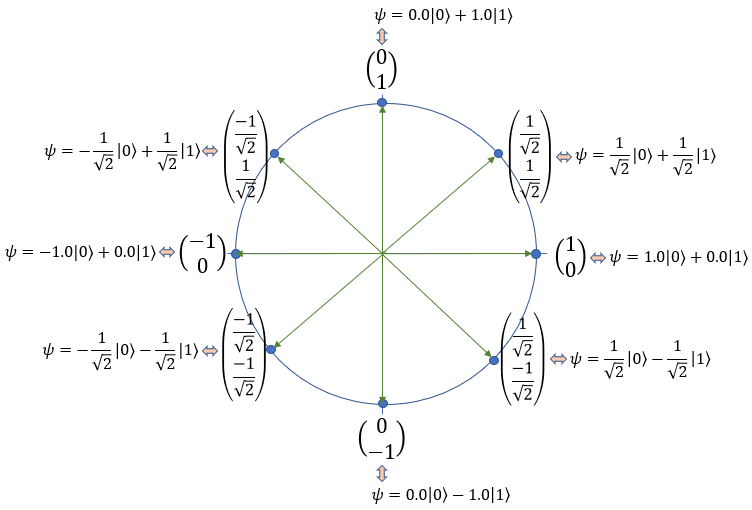2 Qbit Presentation   I hope you got familiar with how to represent single Qbit in mathematical format especially in a vector notation. Then would there be any way to represent two bits in a vector ? There should be. But how ? Is there any generic way to represent the multiple Qbit into a vector ? Yes, there is. The way to represent the multiple qbit into a vector is to multiply multiple single qbit vector with kronecker operator as shown below. For those who is not familiar with kronecker multiplication, refer to my math tutorial on kronecker multiplication / tensor multiplication.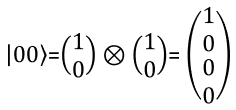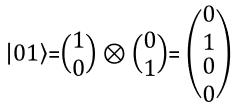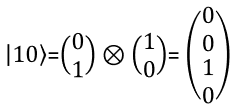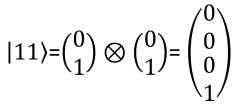The meaning of each numbers in the state vector is interpreted as follows.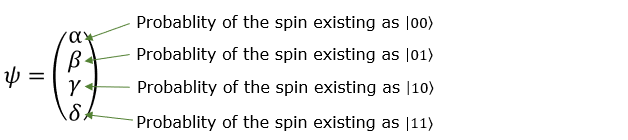This vector can be represented in the form of a probability function as shown below.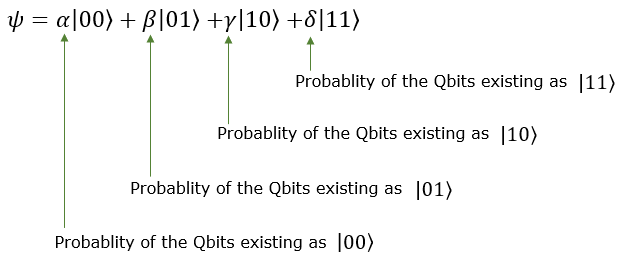Since each of the coefficient indicate the probability for the specific bit combination, following equation should be met.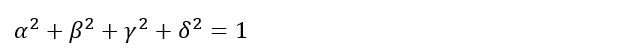3 bit Representation   With the same logic as you present two qbit in a vector, you can represent a three qbit in a vector as shown below. You can extend the same logic to represent N qbits.